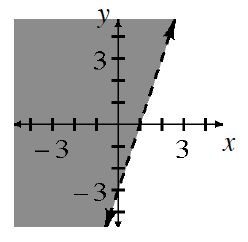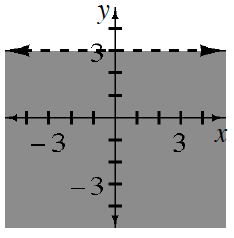### Home > INT3 > Chapter 3 > Lesson 3.2.2 > Problem3-96

3-96.

Sketch the graph of each of the following inequalities on a different set of axes.

1. $3x-3

$y>3x−3$1. $3>y$

$y<3$1. $3x-2y\le6$

Rewrite the inequality as an equation and solve for $y$. Graph the line.

Does the point $(0,0)$ make the original inequality true?

$\textit{y} \ge \frac{3}{2} \textit{x} -2$

1. $x^2-y\le9$

Repeat the steps used in part (a): Solve for $y$; graph the parabola; test $(0,0)$; decide where to shade.

$y\ge x^2−9$